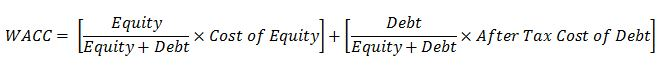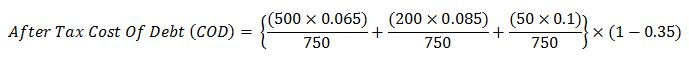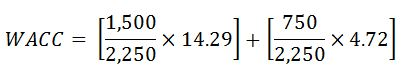# Weighted Average Cost Of Capital (WACC)

Weighted Average Cost Of Capital (WACC) is a calculation of company cost of capital which is based on a weighted average between debt and equity. Its mathematical expression is listed below;WACC represents the minimum return on investment that can be a base line for a company to make a decision for new projects. Generally, companies will accept only projects that have returns more than WACC.

This example will use the previous information from Cost of Debt and Cost of Equity article to demonstrate WACC calculation.Information

James oilfield services issues 500 million \$ in corporate bonds 3 years ago at 6.5 % interest. The company gets a fixed term loan of 200 million \$ at 8.5% interest. Short term debt is 50 million \$ at 10.0% interest. Company corporate tax rate is 35 %. The company has a share price of 50\$/share and this year the company pay investors a dividend of 1.95 \$/share. The company has a strong portfolio in term of work in the future with several oil companies. The company expected dividend growth rate for next year is 10%. The company has a total of 30 million shares.

Total debt = 500 + 200 + 50 = 750 million \$After Tax Cost of Debt (COD) = 4.72 %

## Cost of Equity = [Dc × (1 + G)] ÷ P + G

Where;

Dc = 1.95 \$/share

G = 10 %

P = 50 \$/share

Cost of Equity = [1.95 × (1 + 0. 1)] ÷ 50 + 0.1

Cost of Equity = 0.1429 (14.29 %)

Total Equity = 30 x 50 = 1,500 million \$

Total Equity + Total Debt = 750 + 1,500 = 2,250 million \$WACC =11.1%

Reference BookThomas Sowell (2007) Basic economics: A Common Sense Guide to the Economy, New York, New York, USA: Basic Books.

Share the joy